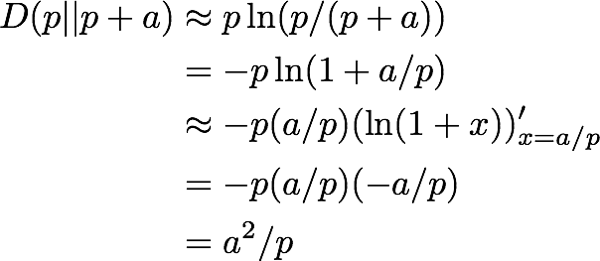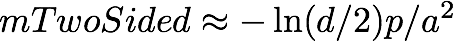# Sample size and power for rare events

We have written a bit on sample size for common events, we have written about rare events, and we have written about frequentist significance testing. We would like to specialize our sample size analysis to rare events (which allows us to derive a somewhat tighter estimate).

In web marketing and a lot of other applications you are trying to estimate a probability of an event (like conversion) where the probability is fairly low (say 5% to 0.5%). In this case we our rules of thumb given in 1 and 2 are a bit inefficient as they do not use that for p near zero on a Bernoulli event we know the variance is necessarily small. Losing that this variance falls with p factor loses a lot of information, so we re-work an estimate (not a bound) that is more aware of p for rare events.

Our claim is: the necessary sample size for an event with probability at least p+a (p,a both positive and small) to appear to have probability at least p with a chance of at least 1-d is about:The derivation is as follows:

From the Chernoff bound we can derive the probability of an event with probability at least p+a showing a frequency below p on a sample of size m is no more than:whereBecause p and a are small we can say:So:Solving for m gives our original claim:Now this isn’t a bound (as in the previous articles) because we used estimates in different steps (and not bounds in steps). But it is a useful because of its extremely simple form.

One thing you can read off this (which you was lost in the earlier estimates) is if you have a = c*p (where c is small constant, i.e. you are trying to measure p to a relative error) then a sample size of -ln(d)/(c^2*p) is appropriate. That is needed sample size does go up as the square of 1/a, but for relative error sample size goes up only linearly in 1/p. In some cases this means constant-dollar sensing is the right strategy for finding good conversion rates. A 1 in 1000 event needs 10 times more samples than a 1 in 100 event, but if it costs a tenth per sample you can afford a large enough sample to measure it also. So choosing between different traffic sources with different conversion rates can be done with a budget that doesn’t depend on the rates to be estimated!

We can demonstrate the simple estimate compared to the Chernoff bound, which is different than the estimate in that it is guaranteed to be at least a large enough sample), and compared to the exact Binomial distribution calculation in R. Lets suppose we want to know what sample size is needed to separate an event of probability 0.04 to have a 95% chance of having a measured frequency of at least 0.036. The R-code is:

```> D <- function(x,y) {
x*log(x/y) + (1-x)*log((1-x)/(1-y))
}
>
> estimateD <- function(lowProb,difference,errorProb) {
-log(errorProb)/D(lowProb,lowProb+difference)
}
>
> print(estimateD(0.036,0.004,0.05))
##  13911.43
>
> estimateT <- function(lowProb,difference,errorProb) {
-log(errorProb)*lowProb/(difference^2)
}
>
> print(estimateT(0.036,0.004,0.05))
##  6740.398
>
>
> errorProbBin <- function(lowProb,difference,size) {
pbinom(ceiling(lowProb*size),size=size,prob=lowProb+difference)
}
>
> library('gtools')
>
> actualSize <- function(lowProb,difference,errorProb) {
r=2*estimateD(lowProb,difference,errorProb)
v=binsearch(function(n) {
errorProbBin(lowProb,difference,n) - errorProb },
range=c(1,r))
v\$where[[length(v\$where)]]
}
>
> print(actualSize(0.036,0.004,0.05))
##  6848
```

What we see is the sample size needed is 6848, the Chernoff bound gives an over-estimate of 13911 and the quick rule of thumb gives 6740 (unreasonably close to the right answer). We can try the same for two sided error:```> estimateTTwo <- function(midProb,difference,errorProb) {
-log(errorProb/2)*midProb/(difference^2)
}
>
> print(estimateTTwo(0.04,0.004,0.05))
##  9222.199
>
> errorProbTwo <- function(midProb,difference,size) {
pbinom(ceiling((midProb-difference)*size),size=size,prob=midProb) +
(1 - pbinom(floor((midProb+difference)*size),size=size,prob=midProb))
}
>
> actualSizeTwo <- function(midProb,difference,errorProb) {
r=2*estimateD(midProb,difference,errorProb)
v=binsearch(function(n) {
errorProbTwo(midProb,difference,n) - errorProb },
range=c(1,r))
v\$where[[length(v\$where)]]
}
>
> print(actualSizeTwo(0.04,0.004,0.05))
##  9432
```

And our two-sided estimate 9222 is fairly close to the actual sample size needed 9432 (but under, which means it is critical to use the actual size, not the estimate to ensure the significance of an experiment).

In this writeup we have only estimated the probability of under-estimates, but we can take the odds of over-estimate as being approximately the same. The idea is: use the rule of thumb to think plan, and then use the exact Binomial probability in R to get exact (one side or two sided) experimental designs.

Edit 2017-05-01: A think you to Nicholas Lepley for pointing out I erroneously had c instead of c^2 in the main result. Sorry about any confusion, and I am grateful for the correction.

Categories: Tutorials### jmount

Data Scientist and trainer at Win Vector LLC. One of the authors of Practical Data Science with R.

1.jmount says: